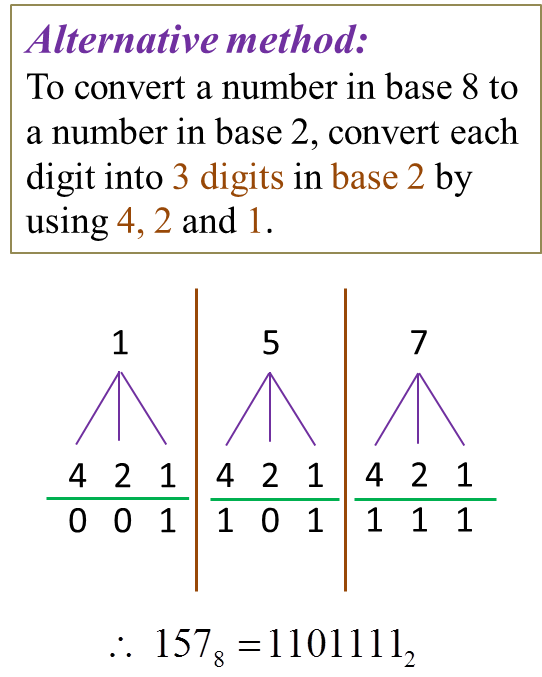# 1.1.3 Numbers in Bases Two, Eight and Five (Part 3)

(E) Converting from One Base to Another
1. The following steps are used to convert a number from one base to another base.

(a)
convert the number to a number in base 10 by using expended notation.

(b)
use repeated division to convert the number in base 10 to the respective bases.

Example 1:
Convert
(a) 1101012 to a number in base 5
(b) 435 to a number in base 2
(c) 3138 to a number in base 5
(d) 4225 to a number in base 8
(e) 1001112 to a number in base 8
(f) 1578 to a number in base 2

Solution:
(a) 1101012
= 1 × 25 + 1 × 24 + 0 × 23+ 1 × 22 + 0 × 21 + 1 × 20
= 5310 ← (Convert from base 2 to base 10)

(b) 435
= 4 × 51 + 3 × 50
= 2310← (Convert from base 5 to base 10)

(c) 3138
= 3 × 82 + 1 × 81 + 3 × 80
= 20310← (Convert from base 8 to base 10)

(d)
4225
= 4 × 52 + 2 × 51 + 2 × 50
= 11210← (Convert from base 5 to base 10)

(e)
1001112
= 1 × 25 + 0 × 24 + 0 × 23+ 1 × 22 + 1 × 21 + 1 × 20
= 3910 ← (Convert from base 2 to base 10)(f) 1578
= 1 × 82 + 5 × 81 + 7 × 80
= 11110← (Convert from base 8 to base 10)Calculator Computation 1. Set the calculator to the ‘BASE’ mode by pressing: [MODE]   [MODE]   [3 (BASE)] 2. Set the calculator to the desired number system by pressing: [BIN] → for base 2 [DEC] → for base 10 [OCT] → for base 8 Key in the following [For (e) and (f) only]: (e) [ BIN ] 100111 [ = ]  [ OCT ] The screen display is:  Therefore 1001112 = 478 (f) [ OCT ] 157 [ = ]  [ BIN ] The screen display is:  Therefore 1578 = 11011112

### 1 thought on “1.1.3 Numbers in Bases Two, Eight and Five (Part 3)”

1.How to express 8^5 as a number base 8 ?